##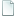Topic: Avoiding zeroes when generating random numbers (Read 4873 times)previous topic - next topic

0 Members and 1 Guest are viewing this topic.
Avoiding zeroes when generating random numbers
##### July 06, 2013, 02:24:12 pm
when using
Code: (javascript) [Select]
`var damage = Math.floor(Math.random()*6); `

for example,

zero also comes up sometimes, is there anyway to get rid of it? Cos' getting zero for damage kinda sucks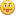Edit: fixed JS tags - radnen

• alpha123
•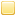Re: Avoiding zeroes when generating random numbers
##### Reply #1 – July 06, 2013, 02:46:38 pm
Code: (javascript) [Select]
`var damage = 1 + Math.floor(Math.random() * 5); // Generate a number `n` such that  0 <= n <= 4 but then add 1 to it`

Code: (javascript) [Select]
`var damage = Math.max(1, Math.floor(Math.random() * 6)); // Pick the larger of 1 and a number `n` (0 <= n <= 5)`

Code: (javascript) [Select]
`var damage = Math.floor(Math.random() * 6) || 1; // 0 is false, so if the random number is 0 that will be `0 || 1` which is 1`

• N E O
••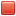Re: Avoiding zeroes when generating random numbers
##### Reply #2 – July 06, 2013, 10:39:46 pm
In all fairness I'd likely only recommend alpha123's first code block since the second and third weigh it so it's twice as likely to pick 1.

• alpha123
•Re: Avoiding zeroes when generating random numbers
##### Reply #3 – July 07, 2013, 01:16:44 am

In all fairness I'd likely only recommend alpha123's first code block since the second and third weigh it so it's twice as likely to pick 1.

Ah, that's true - I didn't think about that. Definitely the first one then.

Too bad Math.ceil(Math.random() * 5) won't work since Math.random() spec'ed to return n where 0 <= n < 1.
Although
Code: (javascript) [Select]
`var damage = Math.ceil(Math.random() * 5) || 1;`
will work.Bah, never mind - that suffers the same bias as the 2nd and 3rd solutions. :/

••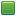• Senior Staff
• Wise Warrior
Re: Avoiding zeroes when generating random numbers
##### Reply #4 – July 07, 2013, 01:58:05 am
It's spec'd to return 0 <= n <= 1 that because it's impossible to get a 0 or 1 with a LCRNG, the excersise is left to the reader.If you use code to help you code you can use less code to code. Also, I have approximate knowledge of many things.

Sphere-sfml here
Sphere Studio editor here

• Flying Jester
•• TurboSphere Developer
Re: Avoiding zeroes when generating random numbers
##### Reply #5 – July 07, 2013, 03:45:15 pm

Code: (javascript) [Select]
`var damage = 1 + Math.floor(Math.random() * 5); // Generate a number `n` such that  0 <= n <= 4 but then add 1 to it`

I always thought that was the standard solution, anyway.

On the other hand, if you are using this for a damage calculation, perhaps having a double weight on a result of 1 wouldn't be a bad thing. It would be a single point of pity-damage, without changing the rest of the distribution. Well, it all depends on what you are trying to do.• alpha123
•Re: Avoiding zeroes when generating random numbers
##### Reply #6 – July 07, 2013, 05:35:56 pm

It's spec'd to return 0 <= n <= 1 that because it's impossible to get a 0 or 1 with a LCRNG, the excersise is left to the reader.That's 0 <= n < 1, but the spec does not care how it's implemented. SpiderMonkey and I believe V8 happen to use LCRNGs, but that's an implementation detail and you shouldn't rely on that. However if that is the case (I don't know much about pseudorandom number generation, so I'll take your word for it) Math.ceil(Math.random() * 5) would work, correct?

••• Senior Staff
• Wise Warrior
Re: Avoiding zeroes when generating random numbers
##### Reply #7 – July 07, 2013, 05:40:53 pm
Oops, yeah you are right. 0 <= n < 1 Silly me,Quick-Soure:
http://en.wikipedia.org/wiki/Linear_congruential_generator
If you use code to help you code you can use less code to code. Also, I have approximate knowledge of many things.

Sphere-sfml here
Sphere Studio editor here

• Fat Cerberus
••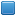• Global Moderator
• miniSphere Developer
Re: Avoiding zeroes when generating random numbers
##### Reply #8 – July 07, 2013, 07:10:38 pm

However if that is the case (I don't know much about pseudorandom number generation, so I'll take your word for it) Math.ceil(Math.random() * 5) would work, correct?

It's been my understanding that you should always implement ranged randoms using floor, regardless of the actual range you need.  Supposedly using either round or ceil will skew the distribution to something other than uniform.

However, there's another caveat: Regardless of whether you use floor, ceil or round, due to floating point rounding issues, there's a very small chance (something on the order of 1 in 2^60 IIRC) that you could get a number one higher than your upper bound.  For that reason, this is the method I use:

Code: (javascript) [Select]
`var rnd = low + Math.min(Math.floor(Math.random() * (high - low + 1)), high)`
miniSphere 5.3.0 - Cell compiler - SSj debugger

• Flying Jester
•• TurboSphere Developer
Re: Avoiding zeroes when generating random numbers
##### Reply #9 – July 07, 2013, 09:09:05 pm

However, there's another caveat: Regardless of whether you use floor, ceil or round, due to floating point rounding issues, there's a very small chance (something on the order of 1 in 2^60 IIRC) that you could get a number one higher than your upper bound.  For that reason, this is the method I use:

Code: (javascript) [Select]
`var rnd = low + Math.min(Math.floor(Math.random() * (high - low + 1)), high)`

Are you sure this applies to JS?

Also, 2^60 is such a small chance (really, really small...) that I wouldn't even bother with that. There's also a chance that HDD errors will corrupt your source code and make the high range one integer higher, that the CPU or RAM will have a hard error (unless you have ECC memory, then there would also have to be an ECC error, which is still possible) and produce a garbage result somewhere, etc. ...I don't think it's really worth putting that into your code.• Fat Cerberus
••• Global Moderator
• miniSphere Developer
Re: Avoiding zeroes when generating random numbers
##### Reply #10 – July 07, 2013, 10:04:26 pm
https://developer.mozilla.org/en-US/docs/Web/JavaScript/Reference/Global_Objects/Math/random

From the top of the Examples section: "Note that as numbers in JavaScript are IEEE 754 floating point numbers with round-to-nearest-even behavior, these ranges, excluding the one for Math.random() itself, aren't exact, and depending on the bounds it's possible in extremely rare cases (on the order of 1 in 2^62) to calculate the usually-excluded upper bound."

Considering that in certain scenarios in Specs (enemy AI, notably, when choosing a random move), I'm using the generated number to index into an array, I figure the extra min() call can't hurt.
miniSphere 5.3.0 - Cell compiler - SSj debugger

• Flying Jester
•• TurboSphere Developer
Re: Avoiding zeroes when generating random numbers
##### Reply #11 – July 07, 2013, 10:13:19 pm
Fair enough. But if I put that into a game, I might be tempted to just let the player instantly win the battle if they got lucky on the order of 1/(2^62)!• Fat Cerberus
••• Global Moderator
• miniSphere Developer
Re: Avoiding zeroes when generating random numbers
##### Reply #12 – July 08, 2013, 12:02:13 am
I'm thorough, what can I say.If it's a bug I can prevent without much effort, I'll account for it.  In fact, the smaller the (nonzero) odds of something like this occurring, the more likely I am to account for it.  Try to reduce the "sufficiently advanced machine" factor, you know?
miniSphere 5.3.0 - Cell compiler - SSj debugger